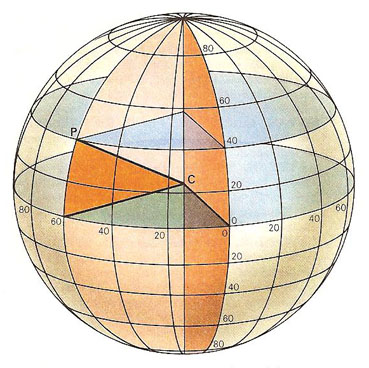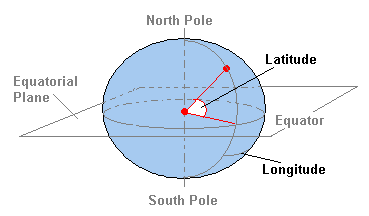A# longitudeMeridians of longitude are great circles passing through the poles and labeled according to their angular distance from a standard meridian – that passing through Greenwich, England. The position of a place on the surface of the Earth can be specified by citing the parallel of latitude and the meridian of longitude which intercept at that place. Here, the coordinates of P are 40°N 60°W, a position in the North Atlantic Ocean some 1,200 km east of New York.Simplified diagram of latitude and longitude. Image: NASA.

Longitude is the angular distance from a point measured east or west along the equator from the prime meridian to the point where the local meridian of the point cuts the equator. Longitude may be expressed either in units of time (hours, minutes, and seconds) or of angle (degrees, minutes, and seconds). It is measured east or west from the prime meridian, through 12 hours or 180°. For convenience in navigation, west longitude is marked plus (+) and east longitude minus (-).

## Longitude at the epoch

The longitude at the epoch is the longitude that a planet has at a given date and date. It is the angle measured along the ecliptic from the vernal equinox eastward to the ascending node, and then eastward along the orbital plane to the planet's position at that instant.

## Longitude of the ascending node

Longitude of the ascending node is an orbital element representing the point where a body crosses the ecliptic (or other orbital reference plane) from south to north.

## Longitude of perihelion

The longitude of perihelion is an angle measured from the vernal equinox eastward along the ecliptic to the ascending node of a planet's orbit, and then continued eastward along the orbital plane to the perihelion. It is one of the orbital elements.

## Solar longitude

Solar longitude is the angular distance along Earth's orbit measured from the intersection of the ecliptic and the celestial equator where the Sun moves from south to north. It gives the position of Earth in its orbit.